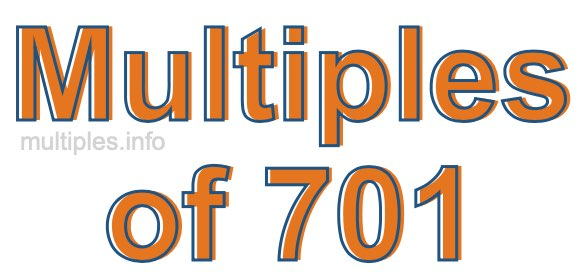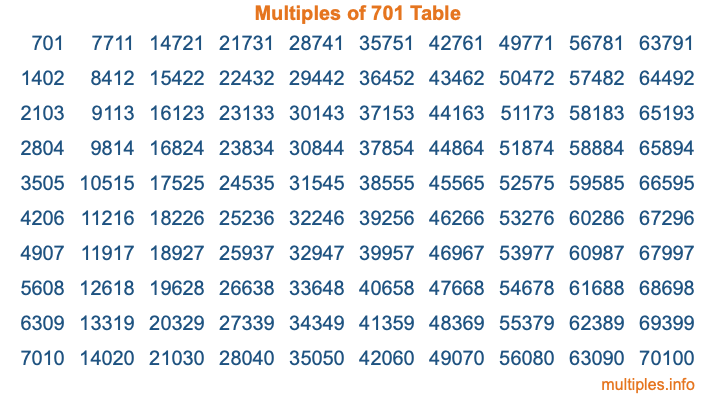Multiples of 701Welcome to the Multiples of 701 page. Here we will first teach you everything you will ever need to know about the multiples of 701, and then give you a study guide summary of everything we taught you to make sure you remember it all. Use this page to look up facts and learn information about the multiples of 701. This page will make you a multiples of seven hundred one expert!

Definition of Multiples of 701
Multiples of 701 are all the numbers that when divided by 701 equal an integer. Each of the multiples of 701 are called a multiple. A multiple of 701 is created by multiplying 701 by an integer.

Therefore, to create a list of multiples of 701, you start with 1 multiplied by 701, then 2 multiplied by 701, then 3 multiplied by 701, and so on for as long as you want. Thus, the list of the first five multiples of 701 is 701, 1402, 2103, 2804, and 3505. To see a larger list of multiples of 701, see the printable image of Multiples of 701 further down on this page. We also have a category where you can choose any nth multiple of 701.

Multiples of 701 Checker
The Multiples of 701 Checker below checks to see if any number of your choice is a multiple of 701. In other words, it checks to see if there is any number (integer) that when multiplied by 701 will equal your number. To do that, we divide your number by 701. If the the quotient is an integer, then your number is a multiple of 701.

Is  a multiple of 701?

Least Common Multiple of 701 and ...
A Least Common Multiple (LCM) is the lowest multiple that two or more numbers have in common. This is also called the smallest common multiple or lowest common multiple and is useful to know when you are adding our subtracting fractions. Enter one or more numbers below (701 is already entered) to find the LCM.

Check out our LCM Calculator if you need more details about the Least Common Multiple or if you need the LCM for different numbers for adding and subtraction fractions.

nth Multiple of 701
As we stated above, 701 is the first multiple of 701, 1402 is the second multiple of 701, 2103 is the third multiple of 701, and so on. Enter a number below to find the nth multiple of 701.

th multiple of 701

Multiples of 701 vs Factors of 701
701 is a multiple of 701 and a factor of 701, but that is where the similarities end. All postive multiples of 701 are 701 or greater than 701. All positive factors of 701 are 701 or less than 701.

Below is the beginning list of multiples of 701 and the factors of 701 so you can compare:

Multiples of 701: 701, 1402, 2103, 2804, 3505, etc.

Factors of 701: 1, 701

As you can see, the multiples of 701 are all the numbers that you can divide by 701 to get a whole number. The factors of 701, on the other hand, are all the whole numbers that you can multiply by another whole number to get 701.

It's also interesting to note that if a number (x) is a factor of 701, then 701 will also be a multiple of that number (x).

Multiples of 701 vs Divisors of 701
The divisors of 701 are all the integers that 701 can be divided by evenly. Below is a list of the divisors of 701.

Divisors of 701: 1, 701

The interesting thing to note here is that if you take any multiple of 701 and divide it by a divisor of 701, you will see that the quotient is an integer.

Multiples of 701 Table
Below is an image of the first 100 multiples of 701 in a table. The table is in chronological order, column by column. The first column has the first ten multiples of 701, the second column has the next ten multiples of 701, and so on.The Multiples of 701 Table is also referred to as the 701 Times Table or Times Table of 701. You are welcome to print out our table for your studies.

Negative Multiples of 701
Although not often discussed or needed in math, it is worth mentioning that you can make a list of negative multiples of 701 by multiplying 701 by -1, then by -2, then by -3, and so on, to get the following list of negative multiples of 701:

-701, -1402, -2103, -2804, -3505, etc.

Multiples of 701 Summary
Below is a summary of important Multiples of 701 facts that we have discussed on this page. To retain the knowledge on this page, we recommend that you read through the summary and explain to yourself or a study partner why they hold true.

There are an infinite number of multiples of 701.

A multiple of 701 divided by 701 will equal a whole number.

701 divided by a factor of 701 equals a divisor of 701.

The nth multiple of 701 is n times 701.

The largest factor of 701 is equal to the first positive multiple of 701.

701 is a multiple of every factor of 701.

701 is a multiple of 701.

A multiple of 701 divided by a divisor of 701 equals an integer.

701 divided by a divisor of 701 equals a factor of 701.

Any integer times 701 will equal a multiple of 701.

Multiples of a Number
Here you can get the multiples of another number, all with the same attention to detail as we did for multiples of 701 on this page.

Multiples of
Multiples of 702
Did you find our page about multiples of seven hundred one educational? Do you want more knowledge? Check out the multiples of the next number on our list!

Copyright  |   Privacy Policy  |   Disclaimer  |   Contact